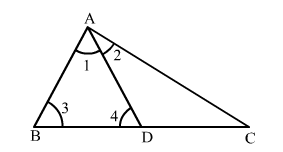# Prove that in a triangle, other than an equilateral triangle,

Question:

Prove that in a triangle, other than an equilateral triangle, angle opposite the longest side is greater than $\frac{2}{3}$ of a right angle.

Solution:Given: In ">ΔABCBC is the longest side.

To prove: $\angle B A C>\frac{2}{3}$ of a right angle, i.e., $\angle B A C>60^{\circ}$

Construct: Mark a point D on side AC such that AD = AB = BD.

Proof:

In ">ΔABD,

"> AD = AB = BD    (By construction)

$\therefore \angle 1=\angle 3=\angle 4=60^{\circ}$

Now

$\angle B A C=\angle 1+\angle 2=60^{\circ}+\angle 2$

but $60^{\circ}=\frac{2}{3}$ of a right angle

So, $\angle B A C=\frac{2}{3}$ of a right angle $+\angle 2$

Hence, $\angle B A C>\frac{2}{3}$ of a right angle.